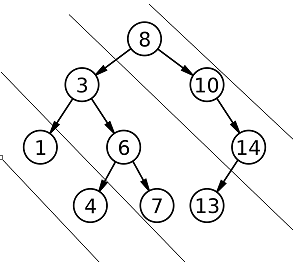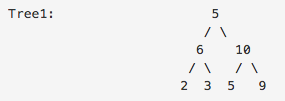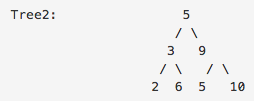2

# Diagonal Anagram

Difficulty: EASY
Avg. time to solve
20 min
Success Rate
80%

Problem Statement
Suggest Edit

#### Diagonals of the binary tree are :#### Diagonal 3 : 1 4

##### For example:
``````For the given binary tree:
````````````Output: True

Explanation: There are 3 diagonals in each tree.
Tree1:
Diagonal 1: 5 10 9
Diagonal 2: 6 3 5
Diagonal 3: 2

Tree2:
Diagonal 1: 5 9 10
Diagonal 2: 3 6 5
Diagonal 3: 2

Since diagonal 1 of tree 1 is an anagram of diagonal 1 of tree 2, similarly diagonal 2 of tree 1 is an anagram of diagonal 2 of tree 2 and similarly with diagonal 3.
Thus, its output should be True.
``````
##### Input Format:
``````The first line of the test case contains elements of the first binary tree in the level order form. The line consists of values of nodes separated by a single space. In case a node is null, we take -1 on its place.

The second line of the test case contains elements of the second binary tree in the level order form. The line consists of values of nodes separated by a single space. In case a node is null, we take -1 on its place.

For example, the input for the tree depicted in the below image would be :
````````````1
2 3
4 -1 5 6
-1 7 -1 -1 -1 -1
-1 -1
``````

#### Explanation :

``````Level 1 :
The root node of the tree is 1

Level 2 :
Left child of 1 = 2
Right child of 1 = 3

Level 3 :
Left child of 2 = 4
Right child of 2 = null (-1)
Left child of 3 = 5
Right child of 3 = 6

Level 4 :
Left child of 4 = null (-1)
Right child of 4 = 7
Left child of 5 = null (-1)
Right child of 5 = null (-1)
Left child of 6 = null (-1)
Right child of 6 = null (-1)

Level 5 :
Left child of 7 = null (-1)
Right child of 7 = null (-1)

The first not-null node (of the previous level) is treated as the parent of the first two nodes of the current level. The second not-null node (of the previous level) is treated as the parent node for the next two nodes of the current level and so on.
The input ends when all nodes at the last level are null (-1).
``````
##### Note :
``````The above format was just to provide clarity on how the input is formed for a given tree.
The sequence will be put together in a single line separated by a single space. Hence, for the above-depicted tree, the input will be given as:

1 2 3 4 -1 5 6 -1 7 -1 -1 -1 -1 -1 -1
``````
##### Output Format:
``````The only line of output will contain “True” or “False”.
``````
##### Note:
``````You are not required to print the output explicitly, it has already been taken care of. Just implement the function.
``````
##### Constraints:
``````0 <= N, M <= 10^5
0 <= node data <= 10^9

Time Limit: 1sec
``````
##### Sample Input 1:
``````1 2 3 -1 -1 -1 -1
3 2 -1 -1
``````
##### Sample Output 1:
``````False
``````
##### Sample Input 2:
``````5 6 10 2 3 5 9 -1 -1 -1 -1 -1 -1 -1 -1
5 3 9 2 6 5 10 -1 -1 -1 -1 -1 -1 -1 -1
``````
##### Sample Output 2:
``````True
``````
##### Explanation to Sample Input 2:
``````There are 3 diagonals in each tree.
Tree1:
Diagonal 1: 5 10 9
Diagonal 2: 6 3 5
Diagonal 3: 2

Tree2:
Diagonal 1: 5 9 10
Diagonal 2: 3 6 5
Diagonal 3: 2
Since diagonal 1 of tree 1 is an anagram of diagonal 1 of tree 2, similarly diagonal 2 of tree 1 is an anagram of diagonal 2 of tree 2 and similarly with diagonal 3.

Thus, its output should be True.
``````